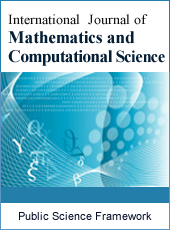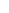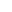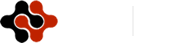International Journal of Mathematics and Computational Science
Articles Information
International Journal of Mathematics and Computational Science, Vol.5, No.2, Jun. 2019, Pub. Date: Jun. 6, 2019
Approximate Solution of Simple Pendulum Equation for Damped and Undamped Oscillatory Motion by Using Homotopy Perturbation Method
 Ahammodullah Hasan, Department of Mathematics, Faculty of Science, Islamic University, Kushtia, Bangladesh.
 Mohammad Masud Rana, Department of Mathematics, Faculty of Science, Islamic University, Kushtia, Bangladesh.
In this article, Homotopy perturbation method (HPM) is applied to find the approximate solution of free oscillation for simple pendulum equation, which is known a well-known nonlinear ordinary differential equation. The Homotopy Perturbation Method deforms a difficult problem into a simple problem which can be easily solved. Firstly, the approximate solution of simple pendulum equation is developed using initial conditions, and then results are compared with the results obtained by numerical solutions. Finally, Homotopy Perturbation Method (HPM) is applied to find the approximate solution of simple pendulum equation with initial conditions. The model absolute error was found below the 5% significance level. The absolute error between homotopy result and numerical result was average 0.0003%. In this study, we have found the Homotopy result and numerical result makes a good agreement. The results reveal that the HPM is very effective, convenient and quite accurate to systems of nonlinear equations. Some examples are presented to show the ability of the method for free oscillations of simple Pendulum equation.
Homotopy Perturbation Method (HPM), Simple Pendulum Equation (SPE), Nonlinear Differential Equation, Damped, Undamped, Approximate Solution, Numerical Solution
 A. Ghorbani and J. S. Nadjafi, “He’s homotopy perturbation method for calculating Adomian’s polynomials,” Int. J. Nonlin. Sci. Numer. Simul., 8, No. 2, 229–332 (2007); https://doi.org/10.1515/IJNSNS.2007.8.2.229.
 J. H. He, “Application of homotopy perturbation method to nonlinear wave equations” Journal of Chaos, Solitons & Fractals, Volume 26, Issue 3, November 2005, Pages 695-700; https://doi.org/10.1016/j.chaos.2005.03.006.
 J. H. He, “Some asymptotic method for strongly”, International Journal of Modern Physics, Vol. 20, No. 10 (2006); 1141–1199; https://www.researchgate.net/publication/228644456.
 M. A. Noor and S. T. Mohyud-Din, “Modified variational iteration method for solving Fisher’s equations”, Journal of Applied Mathematics and Computing, Volume 31, pp. 295–308, (2009), https://link.springer.com/article/10.1007/s12190-008-0212-7.
 M. A. Noor and S. T. Mohyud-Din, “Homotopy perturbation method for solving sixth-order boundary value problems”, Computers & Mathematics with Applications Volume 55, pp. 2953-2972, (2008) https://doi.org/10.1016/j.camwa.2007.11.026.
 M. A. Haji and K. Al-Khaled, “Analytic studies and numerical simulations of the generalized Boussinesq equation”, Applied Mathematics and Computation, Vol. 191,, PP. 320-333, (2007), https://doi.org/10.1016/j.amc.2007.02.090.
 M. A Noor, S. T. Mohyud-Din, “ Homotopy Perturbation Method and Pade Approximants for Solving Flierl-Petviashivili Equation”, Applications and Applied Mathematics: An International Journal, Vol. 3, No. 2, PP. 224-234, (2008), https://www.pvamu.edu/aam/
 J. H. He., “Homotopy perturbation technique”, Computer Methods in Applied Mechanics and Engineering, Vol. 178, Pp. 257-262, (1999), https://doi.org/10.1016/S0045-7825 (99) 00018-3.
 J. H. He., (2000a), “A coupling method of Homotopy technique and a perturbation technique for nonlinear problems”, International Journal of Nonlinear Mechanics, 35, pp. 37-43.
 J. H. He., (1999), “Homotopy perturbation technique computational methods”, Applied Mechanics and Engineering 178, pp. 257-262.
 J. H. He., (2000a), “A coupling method of homotopy technique and a perturbation technique for nonlinear problems”, International Journal of Nonlinear Mechanics 35, pp. 37-43.
 J. H. He., (2003a), “A simple perturbation approach to Blasius equation”, Applied Mathematics and Computation 140, pp. 217-222.
 J. H. He., (2003b), “Homotopy Perturbation Method: A new nonlinear analytical technique”, Applied Mathematics and Computation 135, pp. 73-79.
 J. H. He., (2005), “Application of homotopy perturbation method to nonlinear wave equation”, Chaos Solutions Fractals 26, pp. 295-300.
 J. H. He., (2006). Homotopy perturbation method for solving boundary value problem. Physics Letters A 350, pp. 87-96.
 Ganji DD and Rafei M (2006), “Solitary wave solutions for a generalisezed Hirota- Satsuma coupled Kdv equations by homotopy perturbation method”, Physics Letters A 356, pp. 131-137.
 Biazer JH and Ghazvini (2009), “He’s homotopy perturbation method for solving systems of volterra integral equations”, Chaos Solitons and Fractals 39, pp. 370-377.
 Rafiq A, Hussain S, and Ahmed M (2009), “General homotopy method for Lane-Emden type differrential equations”, International Journal of Applied Mathematics and Mechanics, 5 (3), pp. 75-83.
 Abbasbandy S (2007), “Application of He’s homotopy perturbation method to functional integral equations”, Chasos Solitons and Fractals 31, pp. 1243-1247.
 Wazwaz AM (2004), “The tanh method for travelling wave solutions of nonlinear equations”, Applied Mathematics and Computation, 154, pp. 713–723.
 Gupta, S., Singh, J. and Kumar, D. (2013): Applications of homotopy perturbation transform method for solving time-dependent functional differential equations. International Journal of Nonlinear Science 16 (1), pp. 37–49.
 Mehrabinezhad, M. and Saberi-Nadjafi, J. (2010): Application of He's homotopy perturbation method to linear programming problem, International Journal of Computer Mathematics 88 (2), pp. 341–347.
 Chen, Z. and Jiang, W. (2011): Piecewise homotopy perturbation method for solving linear and nonlinear weakly singular VIE of second order. Applied Mathematics and Computation 217 (19), pp. 7790–7798.
 Ghasemi, M., Tavassoli, M. and Babolian, E. (2007): Numerical solutions of the nonlinear Voltera-Fredholm integral equations by using homotopy perturbation method. Applied Mathematics and Computation 188, pp. 446–449.
 Siddique, A. M., Mahmood, R. and Ghori, Q. K. (2006): homotopy perturbation method for thin film flow of a fourth grade fluid down an inclined plane. Physics Letters A 352 (4–5), pp. 404–410.
 ] Ozis, T. and Yildirim, A. (2007b): Travelling wave solution of KdV equation using He homotopy perturbation method. International Journal of Nonlinear Science and Numerical Simulation 8, pp. 239–242.
 Ozis, T. and Yildirim, A. (2007a): A comparative study of He's homotopy perturbationmethod for determining frequency-amplitude relation of a nonlinear oscillator with discontinuity. International Journal of Nonlinear Science and Numerical Simulation 8 (2), pp. 243–248.
 Rafei, M. and Ganji, D. D. (2006): Explicit solutions of Helmholtz equation and fifth-order KdV equation using homotopy perturbation method. International Journal of Nonlinear Sciences and Numerical Simulation 7, pp. 321–328.
 Ganji, D. D. and Sadighi, A. (2006): Application of He's homotopy perturbation method to nonlinear coupled system of reaction-diffusion equations. International Journal of Nonlinear Sciences and Numerical Simulation 7, pp. 411–418.
 He, J. H. (2000a): A review on some new recently developed nonlinear analytical techniques. International Journal of Nonlinear Sciences and Numerical Simulation 1 (1), pp. 51–70.
 Belendez, A., C. Pascual, M. L. Alvarez, D. I. Mendez and M. S. Yebra (2009), “Higher- order analytical approximate solutions to the nonlinear pendulum by He’s homotopy method”, Vol. 79.
 P. R. Sharma and G. Methi, 2010, Solution of Coupled Nonlinear Partial Differential Equations Using Homotopy Perturbation Method, Department of Mathematics, University of Rajasthan, Jaipur - 302055, India.
 D. D. Ganji, G. Afrouzi, and R. Talarposhti. Application of he’s variational iteration method for solving the reaction-diffusion equation with ecological parameters. Computers & Mathematics with Applications, 54 (7-8): 1010– 1017, 2007.
 D. D. Ganji, M. Nourollahi, and M. Rostamian. A comparison of variational iteration method with adomian decomposition method in some highly nonlinear equations. International Journal of Science & Technology, 2 (2): 179–188, 2007.
 A. Sadighi and D. D. Ganji. Exact solutions of nonlinear diffusion equations by variational iteration method. Computers & Mathematics with Applications, 54 (7-8): 1112–1121, 2007.ISSN Print: 2381-7011
ISSN Online: 2381-702X
Current Issue:
Vol. 5, Issue 2, June Submit a Manuscript Join Editorial Board Join Reviewer Team
 About This Journal All Issues Open Access Indexing Payment Information Author Guidelines Review Process Publication Ethics Editorial Board Peer Reviewers600 ATLANTIC AVE, BOSTON,
MA 02210, USA+001-6179630233
JournalsJournal ListsAuthor GuidelinesPayment InformationSubmit a ManuscriptJoin as Editorial MembersJoin as ReviewersPrivacy NoticeTerms of ConditionsHelp Center